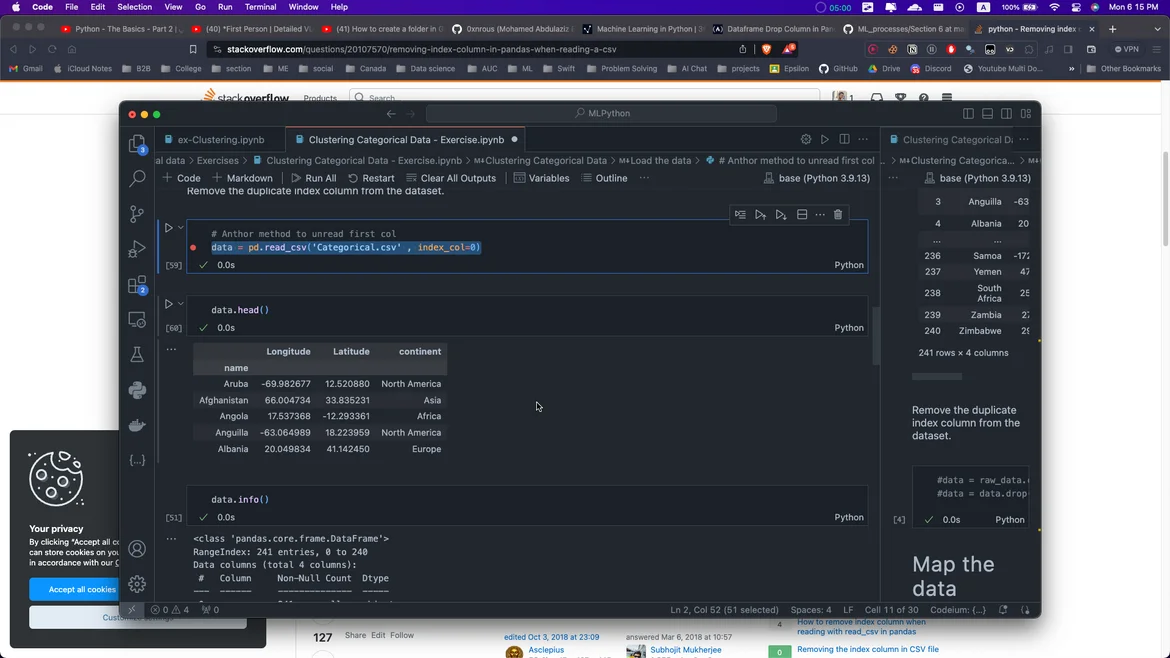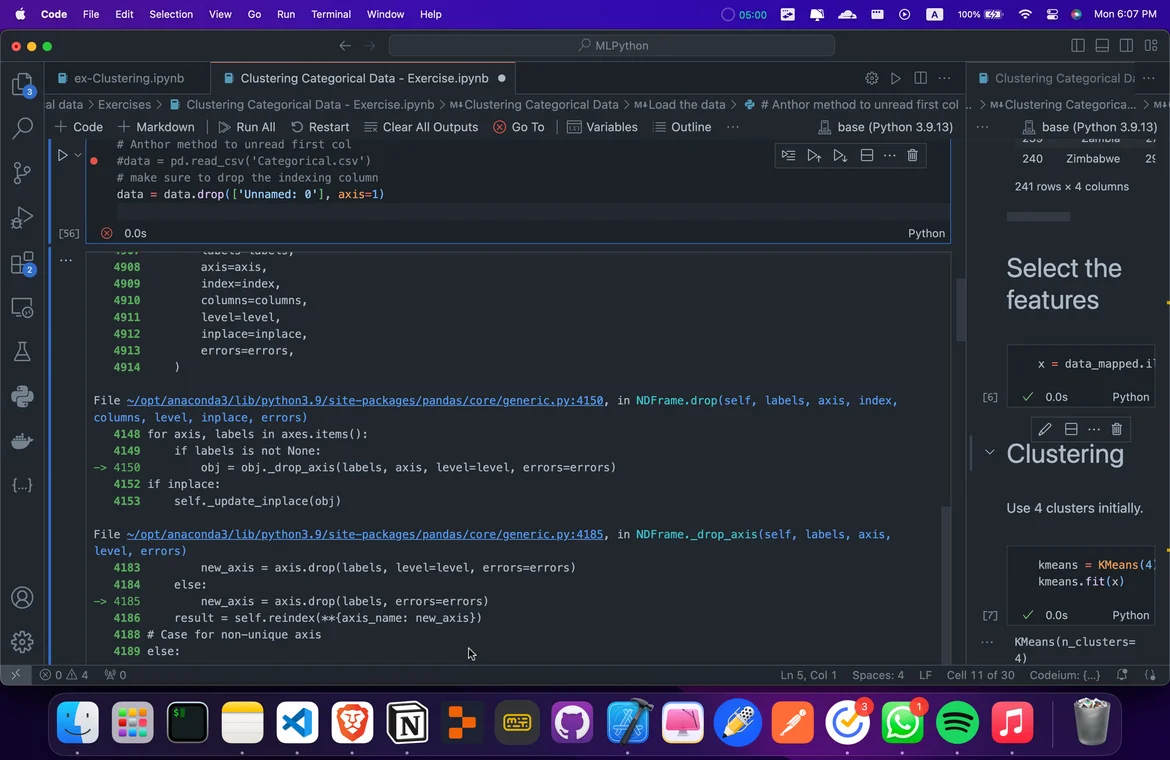10 Oct 2023

Posted on:

09 Oct 2023

0

# Resolved:Exercise for clustering categorical data need to remove index column ........ (I NEED SOLUTION)

I need solve for this i try every thing what problem with this data

second i try >> data.reset_index(drop=True, inplace=True) (not working)

third i try >> data = pd.read_csv('Categorical.csv' , index_col=0) (worked) but when i make some operation not get "continent" as 3 index in dataInstructor
Posted on:

10 Oct 2023

0

Hey Mohamed,

Thanks for reaching out.

The index of a `DataFrame` are the labels that identify each row:

https://pandas.pydata.org/docs/reference/api/pandas.DataFrame.index.html

To the best of my knowledge, you can't remove these identifiers; you can only reset their count, as done in the lecture Linear Regression Practical Example (Part 5).

In fact, you shouldn't need to remove the index column from a `DataFrame`. That would only be necessary if you want to export the `DataFrame` to a CSV file excluding the index column. You can do this by introducing the parameter `index` and setting it to `False`, as follows:

``data.to_csv('test.csv', index = False)``

Hope this helps.

Kind regards,

365 Hristina

Posted on:

10 Oct 2023

0